Simplification Questions with solution for Competitive Exams PDF for free Download. Topic wise Objective MCQ of Maths Simplification topic in Hindi and English. प्रतियोगी परीक्षाओं के लिए सरलीकरण प्रश्न पीडीफ़ मुफ्त डाउनलोड के लिए। हिंदी और अंग्रेजी में गणित सरलीकरण विषय का विषयवार एमसीक्यू।

We are going to share two PDF of Simplification Question and answer with solution and tricks. The questions are selected from the previous year exam question paper of SSC CGL, CHSL, CPO, SSC Constable GD, Banks, UPSSSC, Bank and other government jobs examinations. First PDF contains questions in English and another with same questions in Hindi.

### Simplification Topic Covered

• Type I : BODMAS (Bracket, Of, Division, Multiplication, Addition, Subtraction)
• Type-II: Continued Fraction
• Type – III: Square and Square Root
• Type IV : Cube and Cube Root : Simplification Questions in Hindi

PDF Name :
1) Simplification Questions for Competitive Exams ( English Medium)
2) Simplification Questions with Solution in Hindi ( Hindi Medium)

Details of PDF:
Prepared by : Ajay Gulia, Super Success Institute
Published at : nrastudy.com
Level : SSC, NRA CET, Bank and other Competitive Exams
Number of Pages :
PDF 1 – 7 pages
PDF 2 – 6 pages

### Online Practice of Simplification Questions

Simplification Questions for Competitive Exams – NRA STUDY

Simplification Questions in Hindi with Solution – NRA STUDY

#### Simplification Questions from the above Online Practice set

Q.: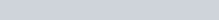$\frac {1}{30} + \frac {1}{42} + \frac {1}{56} + \frac {1}{72} + \frac {1}{90} + \frac {1}{110} = ?$
a)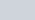$\sqrt {2} \frac {2}{27}$
b)$\frac 19$
c)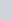$\frac {5}{27}$
d)$\frac{6}{55}$

Ans : d)$\frac{6}{55}$
Solution: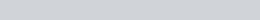$\frac{1}{5 \times 6} + \frac{1}{6 \times 7} + \frac{1}{7 \times 8} + \frac{1}{8 \times 9} + \frac{1}{9 \times 10} + \frac{1}{10 \times 11}$
=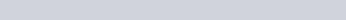$\frac{1}{5} - \frac{1}{6} + \frac{1}{6} - \frac{1}{7} + \frac{1}{7} - \frac{1}{8} + \frac{1}{8} - \frac{1}{9} + \frac{1}{9} - \frac{1}{10} + \frac{1}{10}- \frac{1}{11}$
=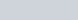$\frac 15 - \frac {1}{11} = \frac {6}{55}$

Q.: 5-[4-{3-(3-3-6)}] is equal to ?
a) 10
b) 6
c) 4
d) 0
Ans : a) 10
Solution: 5-[4-{3-(3-3-6)}] = 5-[4-{3-(-6)}] = 5-[4-{3+6}] = 5-[4-9] =5 -[-5] =10

1.